# AP Calculus AB Practice Test 6

### Test Information10 questions20 minutes

Calculator Disallowed

1. If g(x) =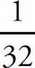x4 - 5x2, find g′(4).

2.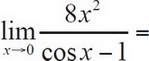3.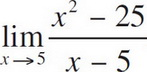is

4. If f(x) =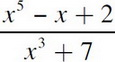, find f′(x).

5. Evaluate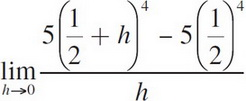.

6.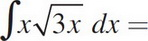7. Find k so that f(x) =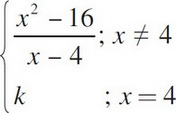is continuous for all x.

8. Which of the following integrals correctly gives the area of the region consisting of all points above the x-axis and below the curve y = 8 + 2x - x2 ?

9. If f(x) = x2 cos 2x, find f′(x).

10. An equation of the line tangent to y = 4x3 - 7x2 at x = 3 is